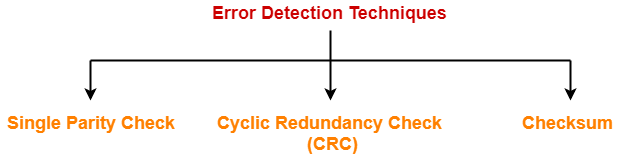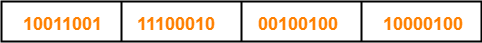## Error Detection in Computer Networks-

 Error detection is a technique that is used to check if any error occurred in the data during the transmission.

Some popular error detection methods are-1. Single Parity Check
2. Cyclic Redundancy Check (CRC)
3. Checksum

## Checksum-

Checksum is an error detection method.

Error detection using checksum method involves the following steps-

## Step-01:

At sender side,

• If m bit checksum is used, the data unit to be transmitted is divided into segments of m bits.
• All the m bit segments are added.
• The result of the sum is then complemented using 1’s complement arithmetic.
• The value so obtained is called as checksum.

## Step-02:

• The data along with the checksum value is transmitted to the receiver.

## Step-03:

• If m bit checksum is being used, the received data unit is divided into segments of m bits.
• All the m bit segments are added along with the checksum value.
• The value so obtained is complemented and the result is checked.

Then, following two cases are possible-

### Case-01: Result = 0

If the result is zero,

• Receiver assumes that no error occurred in the data during the transmission.

### Case-02: Result ≠ 0

If the result is non-zero,

• Receiver assumes that error occurred in the data during the transmission.

## Checksum Example-

Consider the data unit to be transmitted is-

10011001111000100010010010000100

Consider 8 bit checksum is used.

### Step-01:

At sender side,

The given data unit is divided into segments of 8 bits as-Now, all the segments are added and the result is obtained as-

• 10011001 + 11100010 + 00100100 + 10000100 = 1000100011
• Since the result consists of 10 bits, so extra 2 bits are wrapped around.
• 00100011 + 10 = 00100101 (8 bits)
• Now, 1’s complement is taken which is 11011010.
• Thus, checksum value = 11011010

### Step-02:

• The data along with the checksum value is transmitted to the receiver.

### Step-03:

• The received data unit is divided into segments of 8 bits.
• All the segments along with the checksum value are added.
• Sum of all segments + Checksum value = 00100101 + 11011010 = 11111111
• Complemented value = 00000000
• Since the result is 0, receiver assumes no error occurred in the data and therefore accepts it.

## Important Notes-

### Note-01:

• Consider while adding the m bit segments, the result obtained consists of more than m bits.
• Then, wrap around the extra bits and add to the result so that checksum value consists of m bits.

### Note-02:

• While calculating the checksum, if checksum value is needed, then assume it to be zero.
• After calculating the checksum value, substitute the checksum value in the checksum field.

### Note-03:

• The checksum is used in the internet by several protocols although not at the data link layer.

Also Read- Cyclic Redundancy Check (CRC)

## Problem-

Checksum value of 1001001110010011 and 1001100001001101 of 16 bit segment is-

1. 1010101000011111
2. 1011111000100101
3. 1101010000011110
4. 1101010000111111

## Solution-

We apply the above discussed algorithm to calculate the checksum.

• 1001001110010011 + 1001100001001101 = 10010101111100000
• Since, the result consists of 17 bits, so 1 bit is wrapped around and added to the result.
• 0010101111100000 + 1 = 0010101111100001
• Now, result consists of 16 bits.
• Now, 1’s complement is taken which is 1101010000011110
• Thus, checksum value = 1101010000011110

Thus, Option (C) is correct.

To gain better understanding about Checksum Method,

Watch this Video Lecture

Next Article- Access Control Methods | Introduction

Get more notes and other study material of Computer Networks.

Watch video lectures by visiting our YouTube channel LearnVidFun.